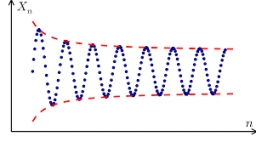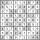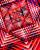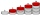# Sum of four numbers

The sum of four consecutive natural numbers is 114. Find them.

a =  27
b =  28
c =  29
d =  30

### Step-by-step explanation:

a+(a+1)+(a+2)+(a+3)=114

4a = 108

a = 27

Our simple equation calculator calculates it.
$b=a+1=27+1=28$
$c=b+1=28+1=29$
$d=c+1=29+1=30$Did you find an error or inaccuracy? Feel free to write us. Thank you!Tips to related online calculators
Do you have a linear equation or system of equations and looking for its solution? Or do you have a quadratic equation?

## Related math problems and questions:

• Even / odd numbersa / Using variable n write two consecutive odd numbers. b / the sum of three consecutive odd numbers is 333. What are this numbers?
• The sumThe sum of five consecutive odd numbers is 75. Find out the sum of the second and fourth of them.
• Simple sequenceContinue with this series of numbers: 1792,448, 112, _, _
• Sum of sevenThe sum of seven consecutive odd natural numbers is 119. Determine the smallest of them.
• Have solutionThe sum of four consecutive even numbers is 92. Determine these numbers.
• QuotientFind quotient before the bracket - the largest divisor 51 a + 34 b + 68 121y-99z-33
• Last pageTwo consecutive sheets dropped out of the book. The sum of the numbers on the sides of the dropped sheets is 154. What is the number of the last page of the dropped sheets?
• Sum of the seventeen numbersThe sum of the 17 different natural numbers is 154. Determine the sum of the two largest ones.
• Digit sumFind the smallest natural number n, the digit sum of which is equal to 37.
• Find unknown 2Find unknown denominator: 2/3 -5/? = 1/4
• Negative differenceFind four arithmetic progression members between 7 and -6.
• LibraryNew books were purchased for the library. Five-eighths were professional books, one-fifth were encyclopedias, and 231 books were dictionaries. How many professional books were there?
• Dropped sheetsThree consecutive sheets dropped from the book. The sum of the numbers on the pages of the dropped sheets is 273. What number has the last page of the dropped sheets?
• The sum 2The sum of five consecutive even integers is 150. Find the largest of the five integers. A.28 B.30 C.34 D.54 Show your solution and explain your answer.
• Find the meanFind the number between 13 and 29.
• Find the sumFind the sum of all natural numbers from 1 and 100, which are divisible by 2 or 5
• Two numbers and its productThe product of two numbers are 2/3. If on of them is 1/10, what is the other?